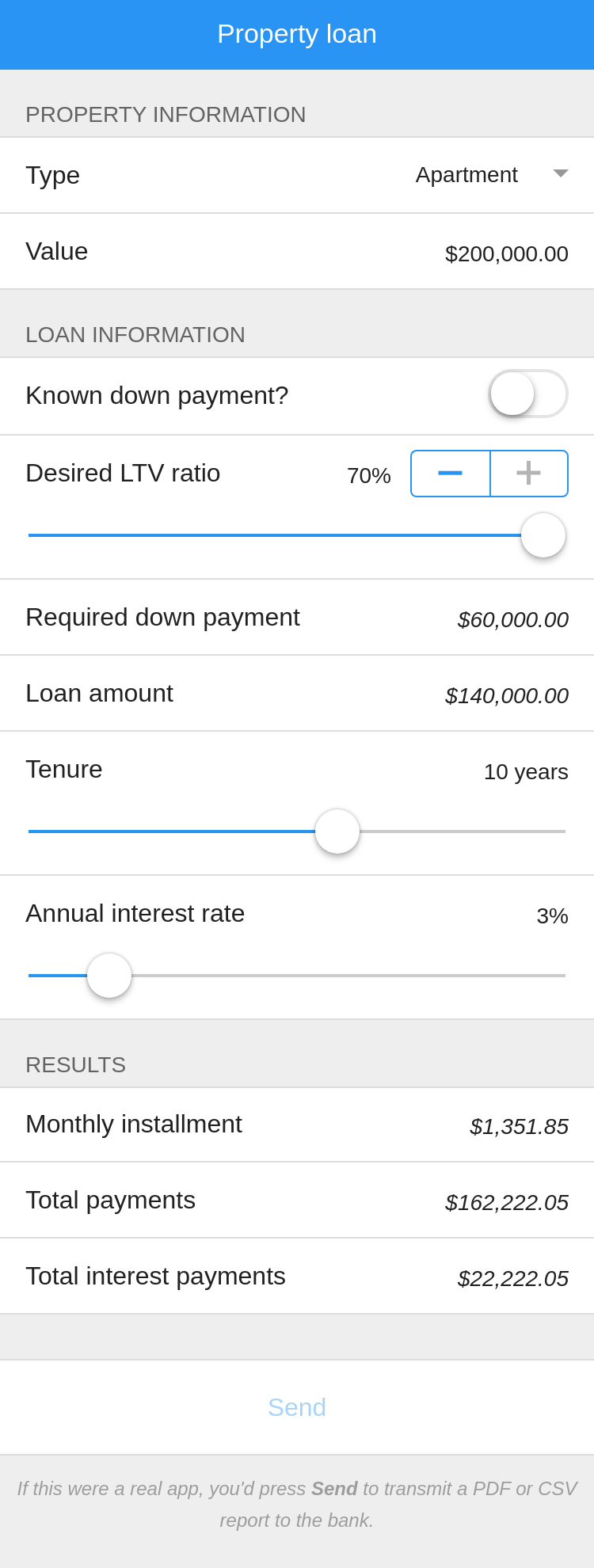• Learn
• Blog
• Pricing

# Sample app: Mortgage calculator

This sample app is concerned with mortgages. The user enters the value of the mortgage, the down payment, the tenure and the annual interest rate. The app then calculates the amount due each month.

One of the most common uses of spreadsheets is to perform calculations related to loans. It’s no wonder, then, that Calcapp is heavily used for this specific purpose.

This sample app is used to calculate mortgages. The user enters the value of the mortgage, the down payment amount, the tenure in years and finally the annual interest rate. The app then calculates the monthly installment (the amount due each month), the total sum of the payments made and the cost of the mortgage.

Here’s a screenshot:Run the app here. This app is available as the Sample: Mortgage template in Calcapp Creator and you are encouraged to experiment with it as you read this blog post.

## The input fields

The first field of the app, Type, is a drop-down field denoting the property type with three values, Apartment, Mini-condo and Condominium. The value selected by users is not part of any calculations, but will be part of any report sent. The Value field holds the value of the property. Initially, it is set to \$200,000.

The Mortgage information group has all other input fields related to the mortgage. The first field, Known down payment? is a switch field, and as a result is either TRUE or FALSE. The user is expected to fill out whether the down payment amount is known.

If the down payment is not known, two fields appear. The Desired LTV ratio is a percentage — LTV stands for Loan To Value. 0% means that the loan will be for the full value of the property with no down payment, 70% means that the loan will only be for 30% of the loan. The Required down payment field then displays the down payment amount using the following formula:

`Value * (1 - DesiredLTVRatio)`

If the down payment is known, the Desired down payment field appears instead, where the down payment amount can be entered directly.

The Desired LTV ratio and Required down payment fields are only shown when the Known down payment? field is FALSE. Consequently, the following formula is associated with their Visible properties:

`KnownDownPayment`

(The ! symbol means “not.” The formula `NOT(KnownDownPayment)` is equivalent.)

Associate a formula with the Visible property by clicking the fx button next to the Visible property in the inspector when the relevant field is selected.

The Desired down payment field is only visible if the Known down payment? field is TRUE. As such, this is the formula associated with its Visible property:

`!KnownDownPayment`

The Mortgage amount field displays the mortgage amount. Its formula needs to subtract the down payment amount from the property value. To determine if the Required down payment field or the Desired down payment field should be used to determine the down payment amount, it needs to consult the Known down payment? field. It does so using the IF formula function:

`IF(KnownDownPayment, Value - DesiredDownPayment, Value - RequiredDownPayment)`

The Tenure field denotes the number of years it takes to fully pay off the mortgage. Type `years` into the Tenure field (to the right of the label) to make that the unit of the field (ensuring that “ years” appears after any number the user enters).

The Annual interest rate field is a percent field, scaling values automatically. That means that if a user enters 15%, the value you’re using in calculations is 0.15. Press the % button in the inspector when the field is selected to make it a percent field.

## The output fields

The Monthly installment field denotes the amount due each month. Its formula uses the PMT formula function, which is an oft-used function in the world of spreadsheets:

`PMT(AnnualInterestRate / 12, Tenure * 12, -MortgageAmount)`

The PMT function returns the periodic payment for a loan. The first parameter is the interest rate and the second parameter is the total number of payments for the loan. The third parameter is the value of the loan (sometimes called the present value or the principal). It is important that all parameters use the same unit, which in this case should be a month.

To provide the monthly interest rate, we need to divide the annual interest rate by 12. Similarly, the total number of payments should be the total number of months, which we get by multiplying the (yearly) tenure by 12. Finally, the loan amount given as the third parameter is made negative. This is done to ensure that the returned result is positive.

There are other parameters to the PMT function which we don’t use here, as we want the mortgage to be fully paid off and payments to be due at the end of every month, not the beginning. To learn more about the PMT function, refer to Calcapp Creator’s reference sidebar.

The Total payments field denotes the total payments made over the course of the mortgage. Its formula simply multiplies the monthly payment by 12 to get the yearly cost, and then multiplies that number with the number of years it takes to pay off the mortgage:

`MonthlyInstallment * 12 * Tenure`

Finally, the Total interest payments field tells you the cost of the mortgage itself. It simply subtracts the mortgage amount from the property value:

`TotalPayments - MortgageAmount`